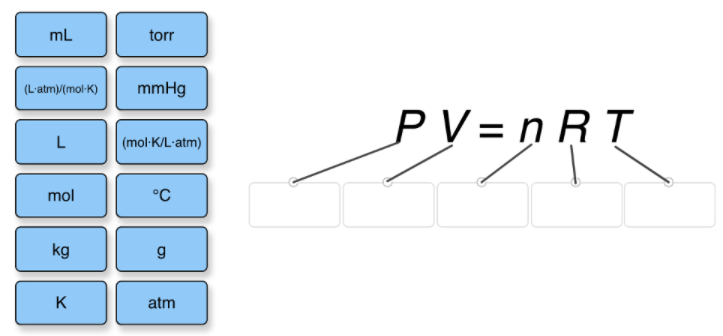# Problem: Label each variable in the ideal gas law with the appropriate, commonly used units

###### FREE Expert Solution

We are asked to label each variable in the ideal gas law with the appropriate, commonly used units

To easily remember the units we simply need to know the units of R.

96% (431 ratings)###### Problem Details

Label each variable in the ideal gas law with the appropriate, commonly used unitsFrequently Asked Questions

What scientific concept do you need to know in order to solve this problem?

Our tutors have indicated that to solve this problem you will need to apply the The Ideal Gas Law concept. You can view video lessons to learn The Ideal Gas Law. Or if you need more The Ideal Gas Law practice, you can also practice The Ideal Gas Law practice problems.